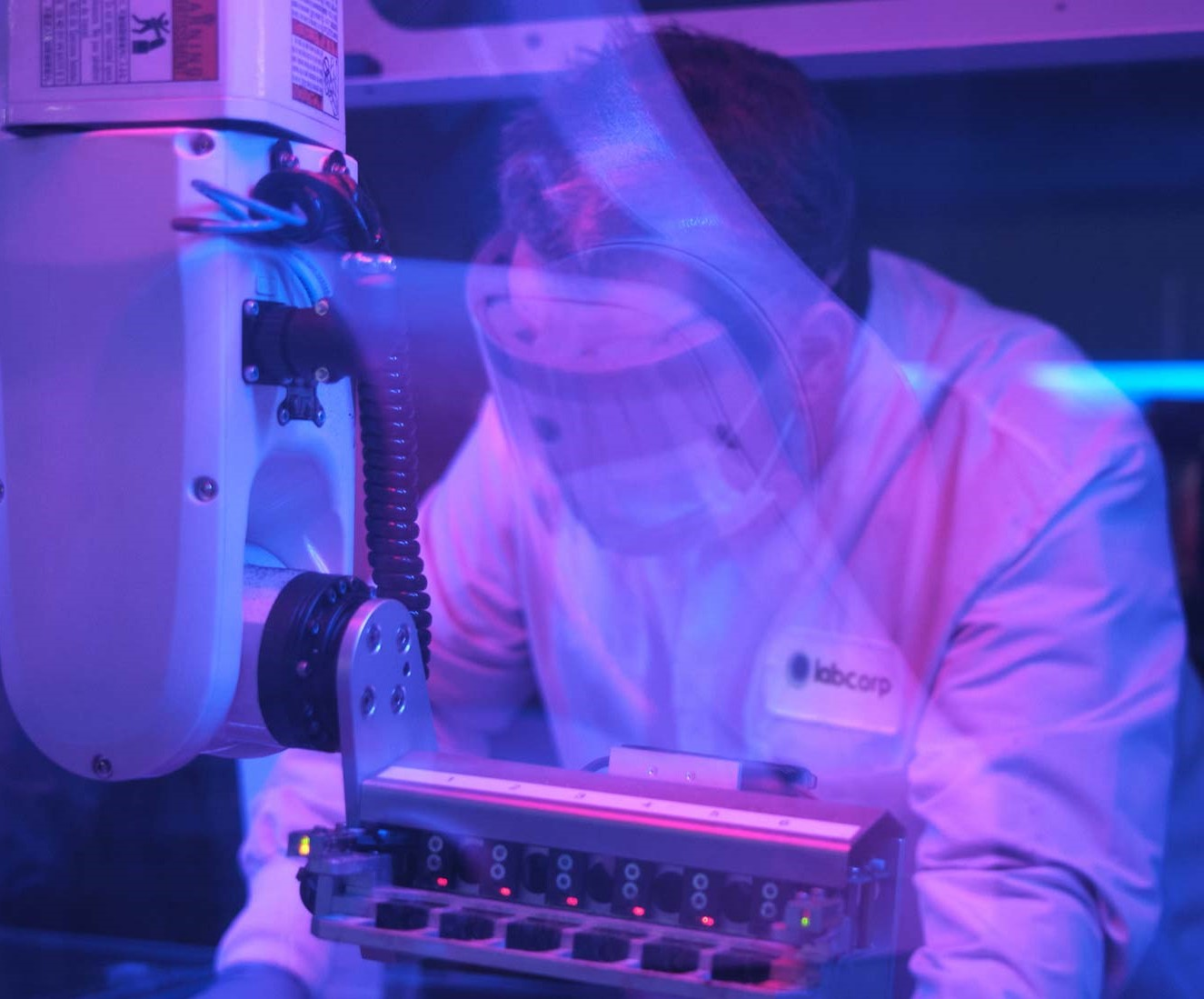LabCorp and its Specialty Testing Group, a fully integrated portfolio of specialty and esoteric testing laboratories.# Plasma Amino Acid Reference Intervals

Analyte

0−30 d

(μmol/L)

31 d−23 m

(μmol/L)

2 y−15 y

(μmol/L)

>15 y

(μmol/L)

Alanine (α-alanine)

121.0−571.0

112.5−500.4

155.8−597.3

124.8−564.2

β-Alanine

1.6−11.8

1.8−9.0

1.2−7.8

1.1−9.0

Alloisoleucine

0.3−2.0

0.3−2.0

0.5−2.5

0.4−3.2

0.0−2.9

0.0−2.6

0.0−1.9

0.0−2.2

γ-Aminobutyric acid (GABA)

0.0−0.6

0.0−0.8

0.0−0.4

0.0−0.3

β-Aminoisobutyric acid

0.1−9.6

0.4−6.4

0.3−3.3

0.3−4.3

α-Amino-N-butyric acid

4.5−31.6

3.9−31.7

6.2−33.5

5.4−34.5

Arginine

19.4−171.0

25.0−140.7

32.7−121.6

32.0−150.0

Argininosuccinic acid

0.0−3.0

0.0−3.0

0.0−3.0

0.0−3.0

Asparagine

20.2−106.6

31.4−100.5

31.6−100.5

29.5−84.5

Aspartic acid

1.8−32.3

1.6−13.4

1.1−8.2

0.9−7.4

Citrulline

4.7−44.3

11.2−48.8

12.0−47.3

13.7−63.2

Cystathionine

0.1−2.1

0.0−0.6

0.0−0.2

0.0−0.7

Cystine

6.1−38.7

5.4−29.4

8.8−36.3

13.5−60.2

Glutamic acid

38.0−398.9

27.0−195.5

18.4−142.2

18.1−155.9

Glutamine

264.0−835.0

280.0−779.0

330.0−726.0

332.0−754.0

Glycine

145.6−518.9

120.0−365.0

129.1−429.7

132.0−467.0

Histidine

42.9−115.2

44.1−106.5

49.8−103.8

47.2−98.5

Homocitrulline

0.0−7.0

0.0−1.3

0.0−1.2

0.0−1.7

Homocystine

0.0−0.1

0.0−0.1

0.0−0.1

0.0−0.1

Hydroxylysine

0.4−3.0

0.3−1.7

0.2−1.0

0.1−0.8

Hydroxyproline

19.0−115.6

9.6−71.4

8.6−45.2

4.7−35.2

Isoleucine

19.3−127.4

25.2−126.4

27.7−110.3

27.7−112.8

Leucine

44.0−224.5

51.1−216.8

56.6−193.6

54.9−205.0

Lysine

83.2−334.2

70.4−279.2

82.7−239.5

94.0−278.0

Methionine

13.9−68.7

11.6−52.0

12.5−40.2

12.7−41.1

Ornithine

30.5−205.7

25.7−131.3

27.3−135.6

30.5−131.4

Phenylalanine

28.5−108.2

30.3−103.3

31.9−103.4

33.6−101.9

Proline

84.3−417.0

79.9−358.3

84.5−365.0

84.8−352.5

Sarcosine

0.0−4.5

0.0−5.4

0.0−4.5

0.0−4.0

Serine

60.6−236.2

65.4−205.6

60.1−171.9

48.7−145.2

Taurine

28.7−238.4

31.1−139.0

33.3−126.0

29.2−132.3

Threonine

60.7−326.8

53.3−262.3

55.9−192.6

67.8−211.6

Tryptophan

20.0−86.0

22.2−95.7

23.9−99.3

23.5−93.0

Tyrosine

21.4−186.9

26.0−140.2

32.2−123.2

31.1−118.1

Valine

73.5−309.4

84.9−345.0

110.0−333.9

102.6−345.4